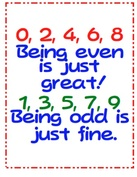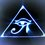# Even, Odd and Fractional Exponents.Continuing my set of notes on exponents here are some things to consider about exponents. If there is a relationship such that $a^{b}=c$ we refer to $a$ as the base, $b$ as the exponent and $c$ as the argument.

If the exponent $b$ is an even number then the argument $c$ will always be positive, whether $a$ is positive or negative.

If the exponent $b$ is an odd number then the argument $c$ will always be positive if $a$ is positve, and negative if $a$ is negative.

If the exponent$b$ is a fraction it results in a radical or "root".

Even roots such as the square root will always be positive. The positive root is called the principal root. This stems from the fact that a negative number times a negative number results in a positive number. Essentially the square roots of negative numbers are imaginary numbers.

Odd roots can be negative or positive. The cube root of a negative number will be a negative number, and the cube root a positive number will be positive.Note by Brody Acquilano
6 years, 3 months ago

This discussion board is a place to discuss our Daily Challenges and the math and science related to those challenges. Explanations are more than just a solution — they should explain the steps and thinking strategies that you used to obtain the solution. Comments should further the discussion of math and science.

When posting on Brilliant:

• Use the emojis to react to an explanation, whether you're congratulating a job well done , or just really confused .
• Ask specific questions about the challenge or the steps in somebody's explanation. Well-posed questions can add a lot to the discussion, but posting "I don't understand!" doesn't help anyone.
• Try to contribute something new to the discussion, whether it is an extension, generalization or other idea related to the challenge.

MarkdownAppears as
*italics* or _italics_ italics
**bold** or __bold__ bold
- bulleted- list
• bulleted
• list
1. numbered2. list
1. numbered
2. list
Note: you must add a full line of space before and after lists for them to show up correctly
paragraph 1paragraph 2

paragraph 1

paragraph 2

[example link](https://brilliant.org)example link
> This is a quote
This is a quote
    # I indented these lines
# 4 spaces, and now they show
# up as a code block.

print "hello world"
# I indented these lines
# 4 spaces, and now they show
# up as a code block.

print "hello world"
MathAppears as
Remember to wrap math in $$ ... $$ or $ ... $ to ensure proper formatting.
2 \times 3 $2 \times 3$
2^{34} $2^{34}$
a_{i-1} $a_{i-1}$
\frac{2}{3} $\frac{2}{3}$
\sqrt{2} $\sqrt{2}$
\sum_{i=1}^3 $\sum_{i=1}^3$
\sin \theta $\sin \theta$
\boxed{123} $\boxed{123}$